﻿ double slit
 Idea of the experiment: Two electrons are emitted from a H2 molecule by photoabsorption. One of these electrons is fast. Since it can emerge from either of the two centers of the H2, it shows double slit interference fringes. The second electron is slow. It interacts with the first electron. This slow electron establishes a minimal environment to the primary electron. The coupling to this second electron leads to decoherence of the primary electron wave resulting in a loss of fringe contrast. By selecting the energy (wavelength) of the second slow electron the degree of decoherence and hence the fringe contrast can be adjusted. The virtue of the experiment is that both electrons are observed in coincidence. This measured two particle momentum distribution unveils that the interference is not lost, but move into the center of mass motion of the two electron. Therefore the fringes in the first electron can be revived by selecting a subsample with fixed relative direction of the second electron. Some Details: What is measured? Double ionization of H2 by circular polarized light: hν + H2 → p + p + e- + e- . Photon energy of 240eV and 160eV, resulting in 20eV Kinetic Energy Release and 190eV (110eV) to be shared between the two electrons. Using COLTRIMS the momentum vector of all four particles is determined with full solid angle. From The direction of the protons the orientation of the molecule (the double slit) is inferred. Both electrons and the molecular axis is measure for each ionization event in coincidence.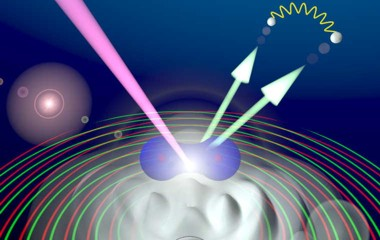Where is the double slit? In a Young type double slit experiment two phase .coherent spherical waves are created by passing a plane wave (e.g. laser light):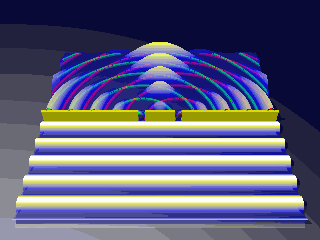Youngs double slit:a plane wave creates two coherentsperical waves which interfere For electrons the same situation can be generated by photoemission of an electron from a either of the two nuclei of homonuclear molecule (H2). The figure shows an electron of 190eV. (This works only if the orbital has pure g (or u) symmetry, because the phase shift between g and u converts fringes to antifringes and hence summing over both destoys the fringe pattern).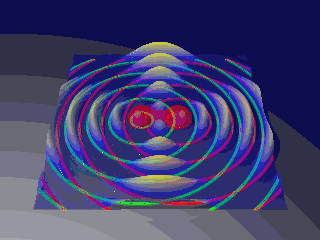Molecular double slit: a circular polarized photon emits two electron waves from either center of the H2 Distribution of the fast electron – switching interference on and off For the fast electron an interference pattern is found only if the second electron.is very slow (has a long wavelength). In this case the angular distribution of the fast electron can be described by the double slit formula.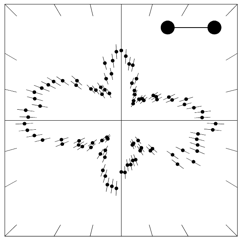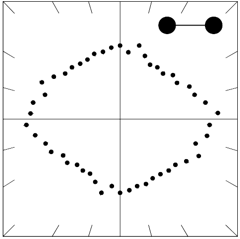left: angular distribution of a 190eV electron from double ionization of H2, when the second electron has 0-5eV right: loss of interference: fast electron 100eV, slow electron 20eV If they have more equal energy the interefence is lost (This is not do to the different wave length of the fast electron, but due to decoherence by electron electron interaction (see paper below for details). Regaining interference If both electron are detected in coincidence the two electron angular distribution unveils why the interference apparently was lost. Depending an the angle between the two electron the interference pattern is rotated.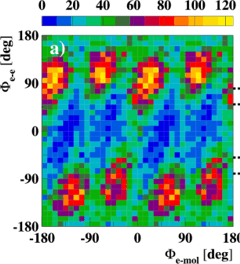View of the complete two electron wave function: (fast electron 100eV slow 20eV) horizontal axis is the angel of the fast electron to the molcuelar axis. The vertical axis shows the angle between the two electron.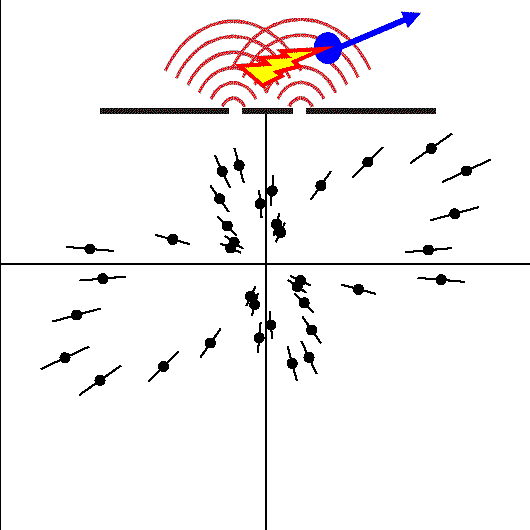Angular distribution of a 100eV electron, second electron has 20eV. The angle between the two electron is fixed to left or right (blue arrow) yielding a rotation of the interference pattern. If the second electron is not obersved this results in the loss of the interference fringes shown above Conclusion: In an open subsystem (one electron in our case) coherence and hence interference can get lost by coupling to the environment. If however the 'environment' (the other electron in our case) is included in the picture there is no decoherence, the quantum features are always present once one obersved the full (closed) system. Recovery of the Quantum Interference in the collective Electron Momentum: The interference which, as discussed above, is lost in the individual electron coordinates by decoherence can be recovered in the momentum of the electron pair. This collective coordinate shows a robust interference pattern which survives the intergration over all other degrees of freedom. This interference can be linked to the emission of both electrons from the same center of the molecule. See PRL 100, 133005 (2008) (PDF 351 kB) for details. View the full text of the Science article (free): The Simplest Double Slit: Interference and Entanglement in Double Photoionization of H2 D. Akoury, K. Kreidi, T. Jahnke, Th. Weber, A. Staudte, M. Schöffler, N. Neumann, J. Titze, L. Ph. H. Schmidt, A. Czasch, O. Jagutzki, R. A. Costa Fraga, R. E. Grisenti, R. Díez Muiño, N. A. Cherepkov, S. K. Semenov, P. Ranitovic, C. L. Cocke, T. Osipov, H. Adaniya, J. C. Thompson, M. H. Prior, A. Belkacem, A. L. Landers, H. Schmidt-Böcking, R. Dörner Science 318, 949 (2007) View the abstract of the Science article View the full text of the Science article Both links above will lead you to the Science Magazine website. If you are unable to access the full text, make sure that you reached this page using exactly the following URL: http://www.atom.uni-frankfurt.de/research/atomic/photonmolecule/H2_double/3_double_slit/. View the full text of the PRL article (free): Interference in the Collective Electron Momentum in Double Photoionization of H2 K. Kreidi, D. Akoury, T. Jahnke, Th. Weber, A. Staudte, M. Schöffler, N. Neumann, J. Titze, L. Ph. H. Schmidt, A. Czasch, O. Jagutzki, R. A. Costa Fraga, R. E. Grisenti, M. Smolarski, P. Ranitovic, C. L. Cocke, T. Osipov, H. Adaniya, J. C. Thompson, M. H. Prior, A. Belkacem, A. L. Landers, H. Schmidt-Böcking, and R. Dörner PRL 100, 133005 (2008) View the PDF file (351 kB) More resources: - German press release - Article in Frankfurter Allgemeine Sonntagszeitung (11.11.2007) (not available online) - Article in Telepolis (german) - Article in Spektrum der Wissenschaft (german) - Article in Physics World (PDF file)